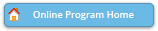#### Abstract Details

 Activity Number: 441 Type: Contributed Date/Time: Tuesday, August 2, 2016 : 2:00 PM to 3:50 PM Sponsor: Section on Bayesian Statistical Science Abstract #321453 Title: Bayesian Approach to Sample Size Determination for Multilevel Logistic Regression Models with Misclassified Outcomes Author(s): Tyler Nelson* Companies: Baylor University Keywords: logistic regression ; Bayesian inference ; Bayesian average power criterion ; identifiability ; misclassification Abstract: We develop a simulation based procedure for determining the required sample size in a multi-level logistic regression model when response data are subject to misclassification. A Bayesian average power criterion, proposed is used to determine a sample size that provides probability of correctly selecting the direction of an association between predictor variables and the probability of event occurrence. Though the model we consider is logistic, the methods proposed could also be used for other count models such as the Poisson. We consider two scenarios, one in which we only have one diagnostic test with misclassification of the response variables, and the second where we will have two independent diagnostic tests in which the response variables are misclassified. Finally we compare the Bayesian power of both scenarios using a simulation approach.

Authors who are presenting talks have a * after their name.